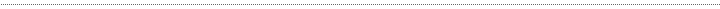Abstract-Codex | index | works | bio |> memory | network | contactTactu5. A Processing music assistant. << back to Tactu5 index Name: T5Notes Example: Note la = new Note( tu.noteToFreq ( T5Notes.LA, 3 ),125,1,1,50,false); Note la2 = new Note(tu.noteToFreq ( T5Notes.LA, 3),250,1,1,90,false); Note la3 = new Note(tu.noteToFreq ( T5Notes.LA, 5 ),250,1,1,100,false); Note la4 = new Note(tu.noteToFreq ( T5Notes.LA, 3 ),250,1,1,200,false); Note la6 = new Note(tu.noteToFreq ( T5Notes.DO, 6 ),250,1,1,200,false); Note la7 = new Note(tu.noteToFreq ( T5Notes.MI, 4 ),200,1,1,100,false); Note la5 = new Note(tu.noteToFreq ( T5Notes.SOL, 5 ),200,1,1,50,false); Description: T5Notes public interface contains two kind of Tactu5 constants. The first ones refer to notes frequency in the 0 octave, for instance these two statements are equivalent: float myFreq = T5Notes.A; float myFreq2= 55.0000; For mantaining formal clarity T5Notes interface provides enharmonic equivalence, so ... float myFreq = T5Notes.F_SHARP; float myFreq2= T5Notes.G_FLAT; .. are the same too. It's possible to use English notation ... float tonic = T5Notes.C; float thirdminor= T5Notes.E_FLAT; float fifth= T5Notes.G; ... as well as Iitalian one. float tonic = T5Notes.DO; float thirdminor= T5Notes.MI_BEMOLLE; float fifth= T5Notes.SOL; French and German notations will be included sometime in the future ( maybe soon). The second type of constants refers to scales and mode structure useful for generating chord and sequence with Tactu5Utility class. They are integers array describing modes intervals in semitones, for example: iint[] minorScale = T5Notes.NATURAL_MINOR; int[] minorScaleStructure = { 2, 1, 2, 2, 1, 2, 2 }; Constants valuelist: Egliash notation: B_SHARP = 32.7032 Hz C = 32.7032 Hz C_SHARP = 34.6478 Hz D_FLAT = 34.6478 Hz D = 36.7081 Hz D_SHARP = 38.8909Hz E_FLAT = 38.8909 Hz E = 41.203453 Hz F_FLAT = 41.2034 Hz E_SHARP = 43.6535 Hz F = 43.6535 Hz F_SHARP = 46.2493 Hz G_FLAT = 46.2493 Hz G = 48.9994f; G_SHARP = 51.9131 Hz A_FLAT = 51.9131 Hz A = 55.0000 Hz A_SHARP = 58.27048 Hz B_FLAT = 58.27048 Hz B = 61.7354 Hz Italian notation SI_DIESIS = 32.7032 Hz DO = 32.7032 Hz DO_DIESIS = 34.6478 Hz RE_BEMOLLE = 34.6478 Hz RE = 36.7081 Hz RE_DIESIS = 38.8909 Hz MI_BEMOLLE = 38.8909 Hz MI = 41.2034 Hz FA_BEMOLLE = 41.2034 Hz MI_DIESIS = 43.6535 Hz FA = 43.6535 Hz FA_DIESIS = 46.2493 Hz SOL_BEMOLLE = 46.2493 Hz SOL = 48.9994 Hz SOL_DIESIS = 51.9131 Hz LA_BEMOLLE = 51.9131 Hz LA = 55.0000 Hz LA_DIESIS = 58.27048 Hz SI_BEMOLLE = 58.27048 Hz SI = 61.7354 Hz Modes and scales MAJOR IONIAN DORIAN PHRYGIAN iLYDIAN MIXOLYDIAN AEOLIAN iNATURAL_MINOR LOCRIAN CHROMATIC WHOLE_TONE OCTATONIC_TONE OCTATONIC_HTONE PENTAPHONIC_MINOR PENTAPHONIC_MAJOR Usage: Web and application. Releated: Sequence ClusterSequence Methods: > chordGenerator() > harmonizer() > noteToFreq() > sequenceGenerator() > transpose() > transposeNotes()Name: chordGenerator() Description: Overwrite a Cluster object. Syntax: tactu5utility.chordGenerator(Note note, int[] mode, int[] modeDegrees, Cluster c); Return: Cluster ^ upName: harmonizer() Description: Sequences harmonizer. Syntax: tactu5utility.harmonizer(Sequence s, int[] mode, int[] modeDegrees, ClusterSequence c) Return: ClusterSequence ^ upName: noteToFreq() Description: Passing a frequency and an octave to transpose this method return a new frequency. Syntax: tactu5utility.noteToFreq(float n, int o) ; Return: float ^ upName: sequenceGenerator() Description: This method generates new sequences contents. Syntax: tactu5utility.sequenceGenerator(Note note, int[] mode, int[] modeDegrees, Sequence s) ; Return: Sequence ^ upName: transpose() Description: This method transpose a frequency for an interval defined in semitone. Syntax: tactu5utility.transpose(float f, float s, int o) ; Return: float ^ upName: transposeNotes() Description: This method transpose all notes included in Sequence and Cluster datatypes for a given interval in semitones. Syntax: tactu5utility.transposeNotes(Sequence s, int s) ; tactu5utility.transposeNotes(Cluster s, int s) ; Return: nothing ^ up<< back^page-up> memory >Tactu5 > T5Notes constants